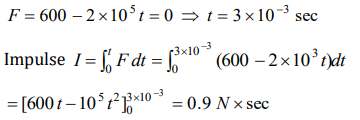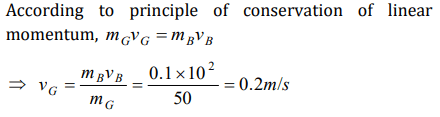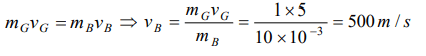## Laws of Motion Questions and Answers Part-16

1. A body of mass M at rest explodes into three pieces, two of which of mass M/4 each are thrown off in perpendicular directions with velocities of 3 m/s and 4 m/s respectively. The third piece will be thrown off with a velocity of
a) 1.5 m/s
b) 2.0 m/s
c) 2.5 m/s
d) 3.0 m/s

Explanation:2. The momentum of a system is conserved
a) Always
b) Never
c) In the absence of an external force on the system
d) None of the above

Explanation: In the absence of an external force on the system

3. A body of mass 0.25 kg is projected with muzzle velocity $100 m s^{-1}$  from a tank of mass 100 kg. What is the recoil velocity of the tank
a) $5 m s^{-1}$
b) $25 m s^{-1}$
c) $0.5 m s^{-1}$
d) $0.25 m s^{-1}$

Explanation: Using law of conservation of momentum, we get
100 * v = 0.25 * 100
v = 0.25 m / s

4. A bullet is fired from a gun. The force on the bullet is given by $F=600-2\times 10^{5}t$    , where F is in newtons and t in seconds. The force on the bullet becomes zero as soon as it leaves the barrel. What is the average impulse imparted to the bullet
a) 9 Ns
b) Zero
c) 0.9 Ns
d) 1.8 Ns

Explanation:5. A bullet of mass 0.1 kg is fired with a speed of 100 m/sec, the mass of gun is 50 kg. The velocity of recoil is
a) 0.2 m/sec
b) 0.1 m/sec
c) 0.5 m/sec
d) 0.05 m/sec

Explanation:6. A bullet mass 10 gm is fired from a gun of mass 1kg. If the recoil velocity is 5 m/s, the velocity of the muzzle is
a) 0.05 m/s
b) 5 m/s
c) 50 m/s
d) 500 m/s

Explanation:7. A rocket can go vertically upwards in earth's atmosphere because
a) It is lighter than air
b) Of gravitational pull of the sun
c) It has a fan which displaces more air per unit time than the weight of the rocket
d) Of the force exerted on the rocket by gases ejected by it

Explanation: Of the force exerted on the rocket by gases ejected by it

8. At a certain instant of time the mass of a rocket going up vertically is 100 kg. If it is ejecting 5 kg of gas per second at a speed of 400 m/s, the acceleration of the rocket would be $\left(g=10 m \diagup s^{2}\right)$
a) $20 m \diagup s^{2}$
b) $10 m \diagup s^{2}$
c) $2 m \diagup s^{2}$
d) $1 m \diagup s^{2}$

Explanation:9. A jet engine works on the principle of
a) Conservation of mass
b) Conservation of energy
c) Conservation of linear momentum
d) Conservation of angular momentum